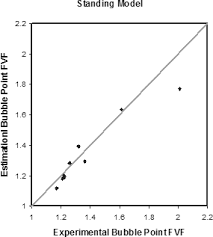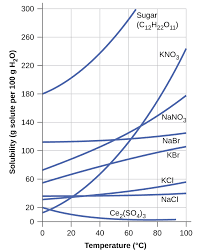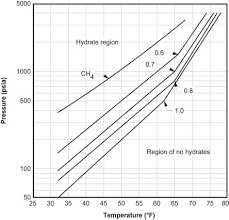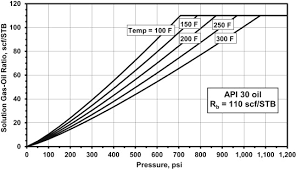## How to Calculate and Solve for Standing Bubble Point Parameter | The Calculator EncyclopediaThe image above represents standing bubble point parameter.

To compute for the standing bubble point parameter, two essential parameters are needed and these parameters are API Gravity (°API) and Temperature (°Rankine) (T).

The formula for calculating standing bubble point parameter:

a = [0.00091(T – 460)] – [0.0125(°API)]

Where:

a = Standing Bubble Point Parameter, a
°API = API Gravity
T = Temperature (°Rankine)

Let’s solve an example;
Find the standing bubble point parameter when API Gravity is 32 and the temperature is 146.

This implies that:

°API = API Gravity = 32
T = Temperature (°Rankine) = 146

a = [0.00091(T – 460)] – [0.0125(°API)]
a = [0.00091(146 – 460)] – [0.0125(32)]
a = [0.00091(-314)] – [0.0125(32)]
a = [-0.28574] – [0.0125(32)]
a = [-0.28574] – [0.4]
a = -0.68574

Therefore, the standing bubble point parameter, a is -0.68574.

## How to Calculate and Solve for Glass Gas Solubility in a Fluid | The Calculator EncyclopediaThe image above represents glass gas solubility.

To compute for the glass gas solubility, four essential parameters are needed and these parameters are API Gravity (°API), Temperature (°Rankine) (T), Mean Bubble Point (Pb*) and Gas Gravity at Actual Separator Psep and Tsepg).

The formula for calculating the glass gas solubility:

γgs = γg[((°API)0.989 / (T – 460)0.172)Pb*]1.2255

Where:

γgs = Glass Gas Solubility
°API = API Gravity
T = Temperature (°Rankine)
Pb* = Mean Bubble Point
γg = Gas Gravity at Actual Separator Psep and Tsep

Let’s solve an example;
Find the glass gas solubility when the API Gravity is 15, the temperature is 30, the mean bubble point is 40 and the gas gravity at actual separator is 54.

This implies that;

°API = API Gravity = 15
T = Temperature (°Rankine) = 30
Pb* = Mean Bubble Point = 40
γg = Gas Gravity at Actual Separator Psep and Tsep = 54

γgs = γg[((°API)0.989 / (T – 460)0.172)Pb*]1.2255
γgs = 54[((15)0.989 / (30 – 460)0.172)40]1.2255
γgs = 54[((15)0.989 / (-430)0.172)40]1.2255
γgs = 54[((15)0.989 / (NaN))40]1.2255
γgs = 54[(14.5 / NaN)40]1.2255
γgs = 54[(NaN)40]1.2255
γgs = 54[NaN]1.2255
γgs = 54[NaN]
γgs = NaN

Therefore, the glass gas solubility is NaN.

## How to Calculate and Solve for Separator Gas Gravity in a Fluid | The Calculator EncyclopediaThe image above represents separator gas gravity.

To compute for the separator gas gravity, four essential parameters are needed and these parameters are API Gravity (°API), Actual Separator Temperature (°Rankine) (Tsep), Actual Separator Pressure (Psep) and Gas Gravity at Actual Separator Psep and Tsepg).

The formula for calculating the separator gas gravity:

γgs = γg[1 + 5.912(10-5)(°API)(Tsep – 460)log(Psep / 114.7)]

Where:

γgs = Separator Gas Gravity
°API = API Gravity
Tsep = Actual Separator Temperature (°Rankine)
Psep = Actual Separator Pressure
γg = Gas Gravity at Actual Separator Psep and Tsep

Let’s solve an example;
Find the separator gas gravity with an API Gravity of 21, actual separator temperature of 18, actual separator pressure of 14 and gas gravity at actual separator of 32.

This implies that;

°API = API Gravity = 21
Tsep = Actual Separator Temperature (°Rankine) = 18
Psep = Actual Separator Pressure = 14
γg = Gas Gravity at Actual Separator Psep and Tsep = 32

γgs = γg[1 + 5.912(10-5)(°API)(Tsep – 460)log(Psep / 114.7)]
γgs = 32[1 + 5.912(10-5)(21)(18 – 460)log(14 / 114.7)]
γgs = 32 [1 + 5.912(10-5)(21)(18 – 460) log(0.1220)]
γgs = 32 [1 + 5.912(10-5)(21)(-442) log(0.1220)]
γgs = 32 [1 + 5.912(10-5)(21) (-442) (-0.9134)]
γgs = 32 [1 + 0.501]
γgs = 32 [1.501]
γgs = 48.039

Therefore, the separator gas gravity is 48.039.

## How to Calculate and Solve for Vasquez-Beggs Gas Solubility in a Fluid | The Calculator EncyclopediaThe image above represents the Vasquez-Beggs Gas Solubility.

To compute for the Vasquez-Beggs gas solubility, five essential parameters are needed and these parameters are API Gravity (°API), Temperature (°Rankine) (T), Gas Gravity at Reference Separator Pressure (γgs), Gas Gravity at Actual Separator Psep and Tsep g) and Gas Solubility Parameter (C1, C2, C3).

The formula for calculating Vasquez-Beggs gas solubility:

Rs = C1 γgs γgC2 exp[C3(°API / T)]

Where:

Rs = Vasquez-Beggs Gas Solubitity
°API = API Gravity
T = Temperature (°Rankine)
γgs = Gas Gravity at Reference Separator Pressure
γg = Gas Gravity at Actual Separator Psep and Tsep
C1, C2, C3 = Gas Solubility Parameter

Let’s solve an example;
Find the Vasquez-Beggs gas solubility when the API Gravity is 24, Temperature is 180, gas gravity at reference separator pressure is 60, gas gravity at actual separator is 56, gas solubility parameter is 72, 66 and 45.

This implies that;

°API = API Gravity = 24
T = Temperature (°Rankine) = 180
γgs = Gas Gravity at Reference Separator Pressure = 60
γg = Gas Gravity at Actual Separator Psep and Tsep = 56
C1, C2, C3 = Gas Solubility Parameter = 72, 66, 45

Rs = C1 γgs γgC2 exp[C3(°API/ T)]
Rs = 72 x 60 x 5666 exp[45(24/ 180)]
Rs = 72 x 60 x 5666 exp[45(0.133)]
Rs = 72 x 60 x 5666 exp
Rs = 72 x 60 x 2.401e+115 exp
Rs = 72 x 60 x 2.401e+115 x 403.428
Rs = 6.974e+119

Therefore, the Vasquez-Beggs gas solubility is 6.974e+119.